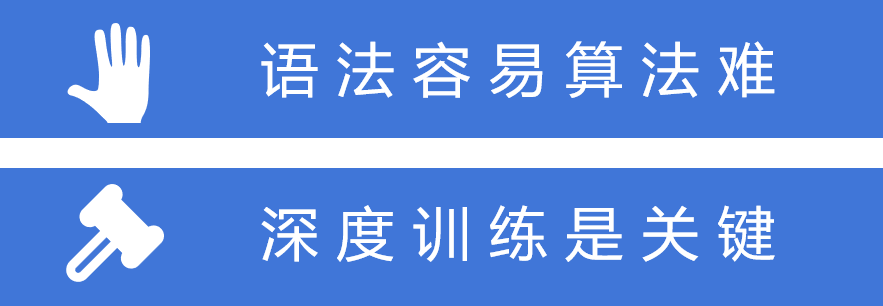集算器 - 高性能计算数据库

集算器是什么

• 一款专用的高性能计算数据库，简称：SPL Base
• SPL（Structured Process Language）是集算器内置的程序语言
• 高性能是集算器的主要特征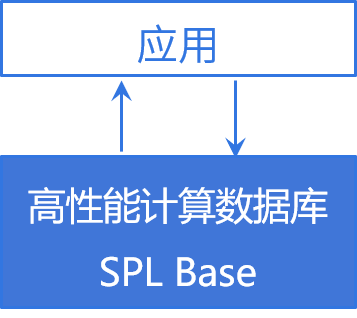为什么现有技术跑不快

计算性能的决定性因素

• 计算效率取决于硬件和软件两方面
• 软件性能就是算法效率
• 算法效率由算法设计和算法实现共同决定
• 好算法只想得出，实现不了也是徒劳
• 缺乏高性能机制的编程语言会限制好算法的实现

结构化数据是重点

• 当前数据计算仍以业务系统产生的结构化数据为主
• 业界在提升结构化数据计算性能时主要依靠大内存、大集群
• 大内存、大集群的实质是纵向或横向提升硬件能力
• 而软件核心仍然使用SQL为主的关系代数体系
• SQL无法实现高性能算法

SQL无法实现高性能结构化数据计算

SQL的问题

• SQL难以实现高效算法的原因在于其理论体系（关系代数）
• 理论上的缺陷很难通过工程实现来弥补
【举例】1亿行数据取前10名在SQL下会怎么做？
• SQL理论上会将所有数据排序，然后取前10名，效率很低
• 大家都知道有不必大排序的办法实现这个运算，却无法用SQL表达
• 只能用指望数据库引擎自动优化，但复杂情况时数据库并不会优化

关于JAVA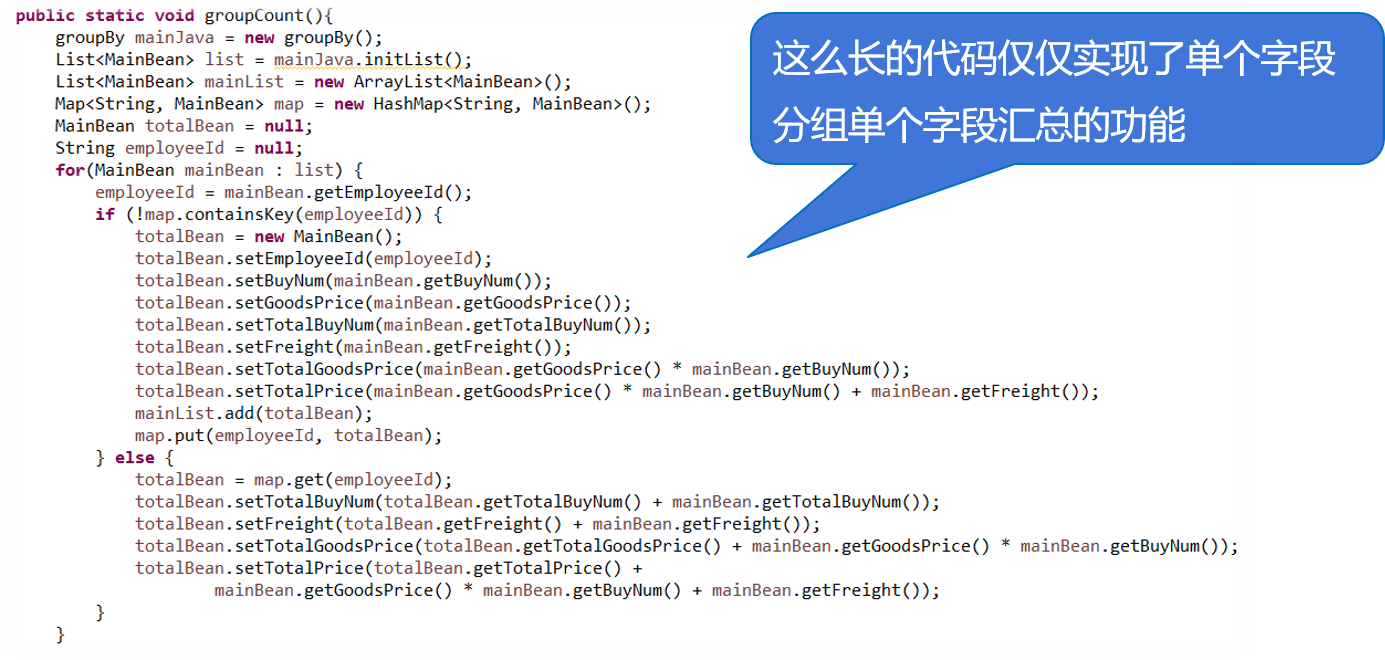高性能的本质集算器为什么跑得快

集算器高性能来自于创新计算体系

【类比】计算1+2+3+…+100=?

1+2=3
3+3=6
6+4=10
10+5=15
15+6=21

1+100=101
2+99=101

50*101=5050

• 集合化
• 离散性
• 深度集合化
• 有序性

敏捷语法更简单

select max(continuousDays)-1
from (select count(*) continuousDays
from (select sum(changeSign) over(order by tradeDate) unRiseDays
case when closePrice>lag(closePrice) over(order by tradeDate)
then 0 else 1 end changeSign
from stock) )
group by unRiseDays)

SQL解法

SQL在使用窗口函数的情况下嵌套三层完成；

A
2 =0
3 =A1.max(A2=if(closePrice>closePrice[-1],A2+1,0))

• 二分法
• 序号定位
• 位置索引
• 哈希索引
• 多层序号定位

• 文本文件的分段
• 集文件及倍增分段
• 数据类型
• 组表与列存
• 有序与补文件
• 数据更新及复组表

• 二分法
• 哈希索引
• 排序索引
• 行存和带值索引
• 索引预加载
• 批量查找
• 返回集合的查找
• 多索引归并
• 全文检索

• 延迟游标
• 遍历复用
• 并行遍历
• 数据库并行加载
• 多路游标
• 分组汇总
• 聚合理解
• 冗余分组键

• 有序分组汇总
• 有序分组子集
• 程序游标
• 前半序分组
• 后半序分组
• 序号分组与可控分段
• 索引排序

• 外键地址化
• 临时地址化
• 外键序号化
• 内连接语法
• 索引复用
• 对位序列
• 大维表查找
• 单边分堆

• 有序归并
• 分段归并
• 关联定位
• 附表

• 部分预汇总
• 时间段预汇总
• 冗余排序
• 对位序列
• 标签位维度
• 内存标签异动

• 计算与数据分布
• 集群复组表
• 复写维表
• 分段维表
• 冗余式容错
• 备胎式容错
• 多作业负载均衡

高性能算法举例

聚合理解

A
1 =file("data.ctx").create().cursor()
2 =A1.groups(;top(10,amount)) 金额在前10名的订单
3 =A1.groups(area;top(10,amount)) 每个地区金额在前10名的订单

遍历复用

A
1 =file("order.ctx").create().cursor() 准备遍历
2 =channel(A1).groups(product;count(1):N) 配置复用计算
3 =A1.groups(area;sum(amount):amount) 遍历，并获得分组结果
4 =A2.result() 取出复用运算的结果

高性能存储

集文件

• 倍增分段方式支持任意数量并行
• 自有高效压缩编码（减少空间；CPU占用少；安全）
• 泛型存储，允许集合数据

组表

• 行列混合存储
• 有序存储提高压缩率和定位性能
• 高效智能索引
• 倍增分段方式支持任意数量并行
• 主子表合一减少存储与关联
• 排号键值实现高效定位关联

分布式计算

数据容错和计算容错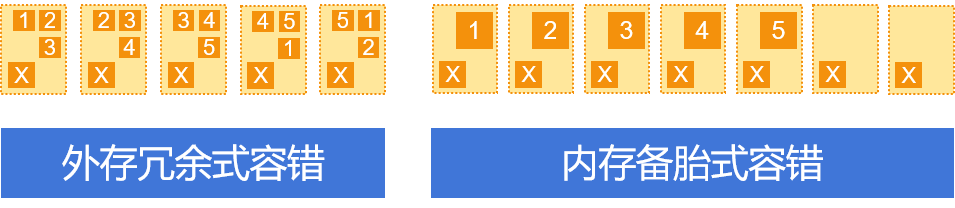集算器性能表现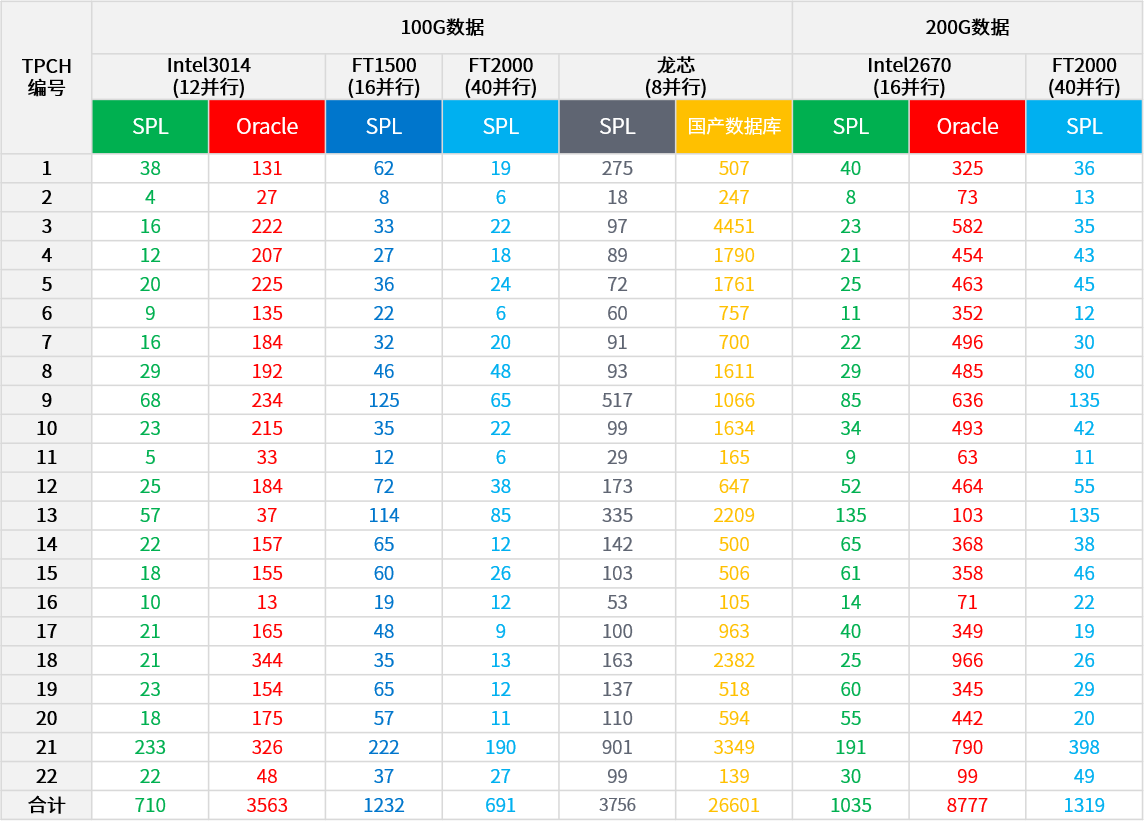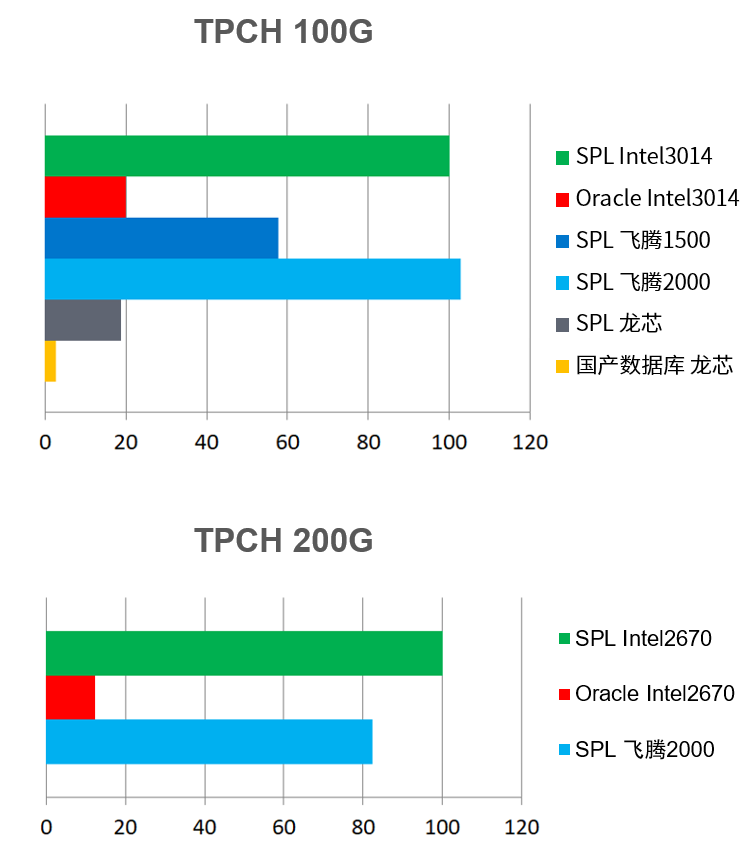* Intel3014 1.7G/12核/64G内存 - 飞腾FT1500/16核/32G内存 - 龙芯/8核/64G内存 - Intel2670 2.6G/16核/128G内存 - 飞腾FT2000/64核/256G内存

应用构架与场景

应用架构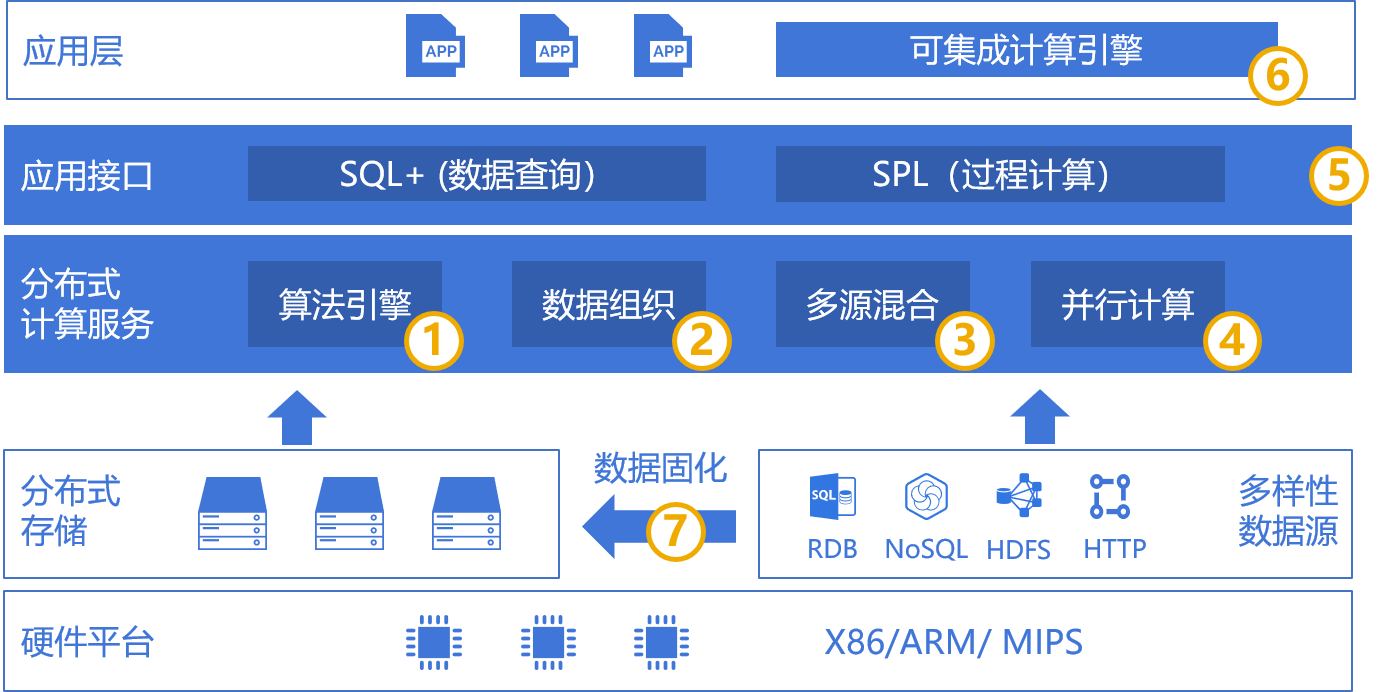• 在线查询统计
• 交互分析取数
• 离线定时跑批

在线统计查询

【场景特征】多并发，业务可能复杂，秒级响应，大数据需集群支持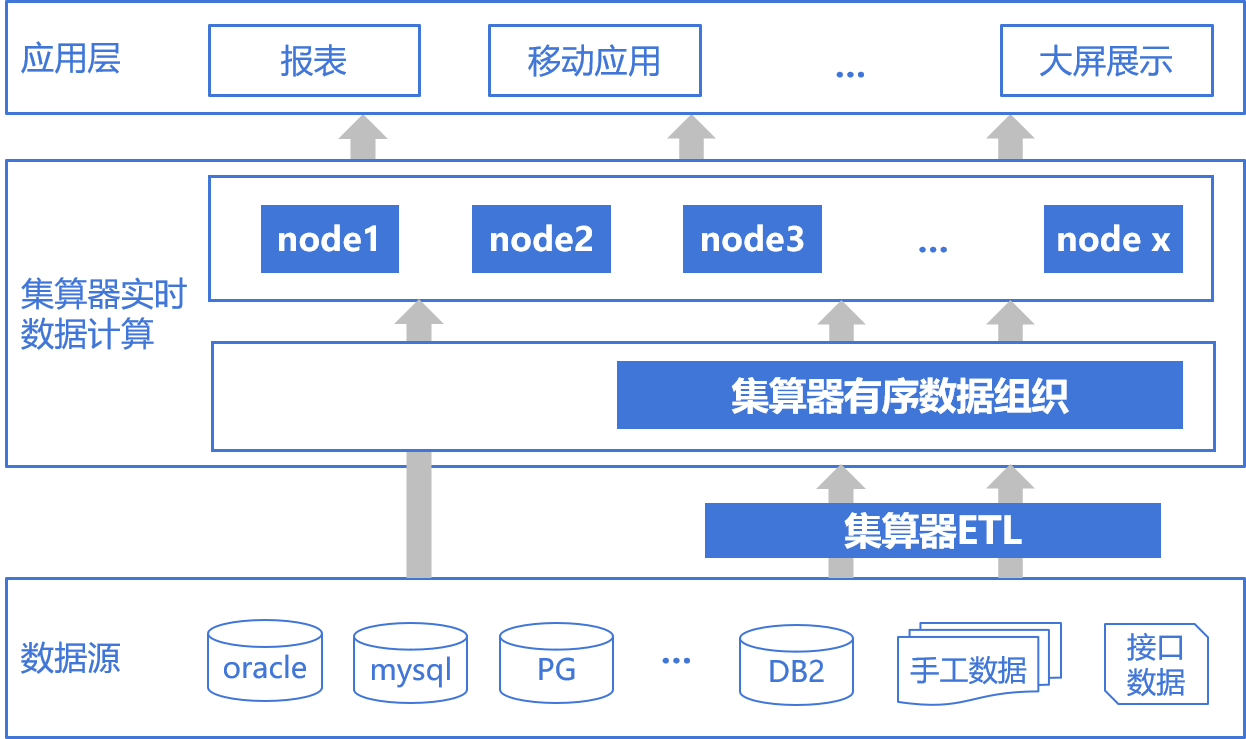交互分析取数

【场景特征】无并发，实时性要求高，会根据上一步的计算结果决定下一步计算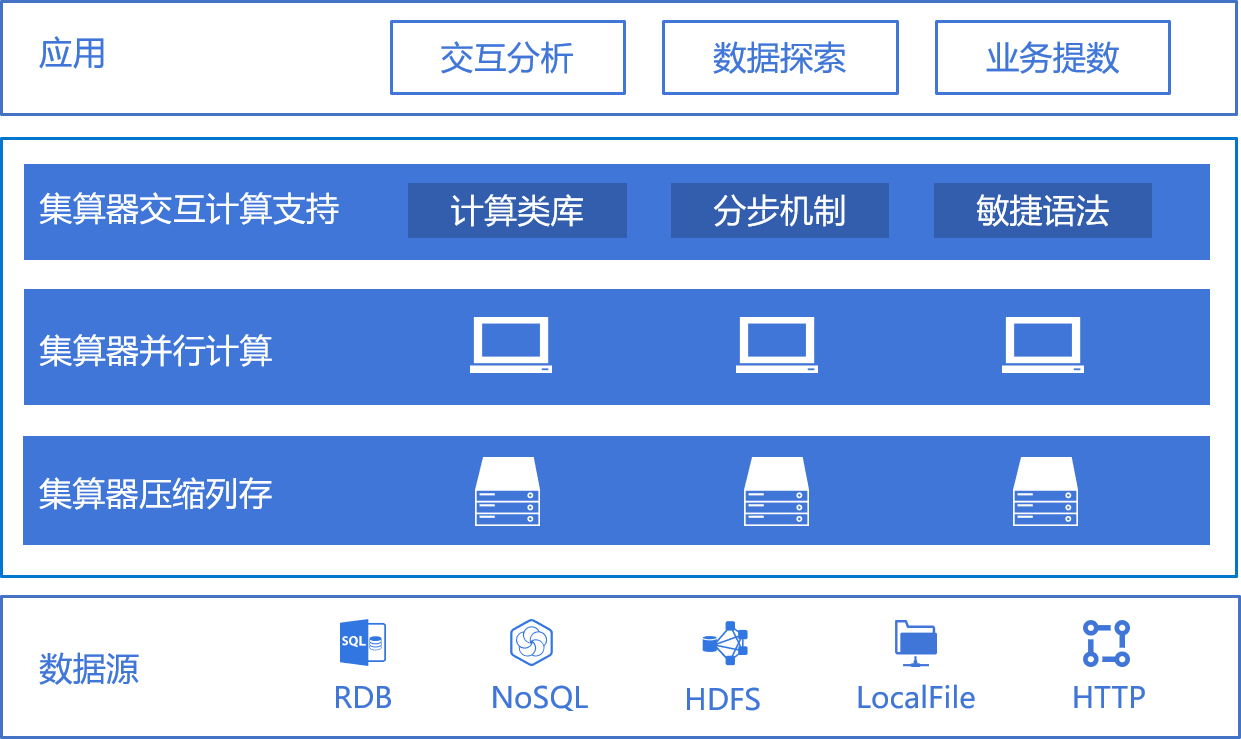离线定时跑批

【场景特征】无并发，不必实时，数据量巨大，对时间窗口要求高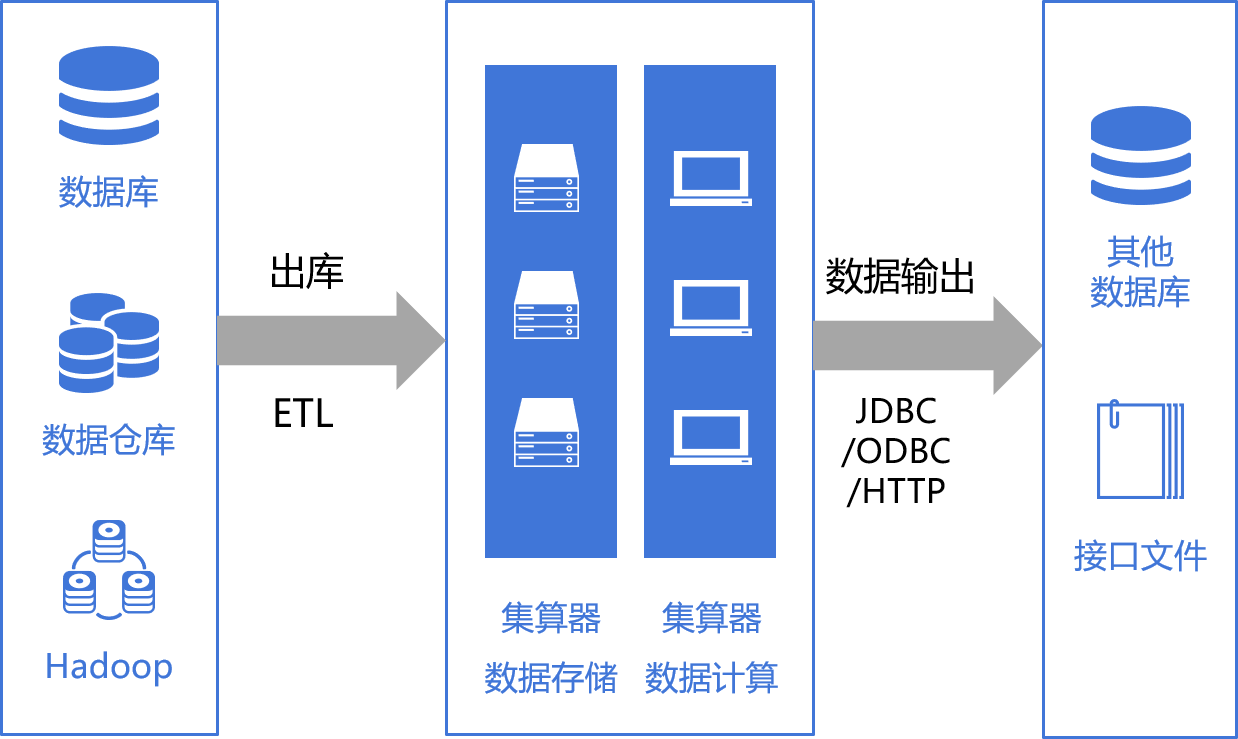高性能常见问题

集算器要自行存储数据吗?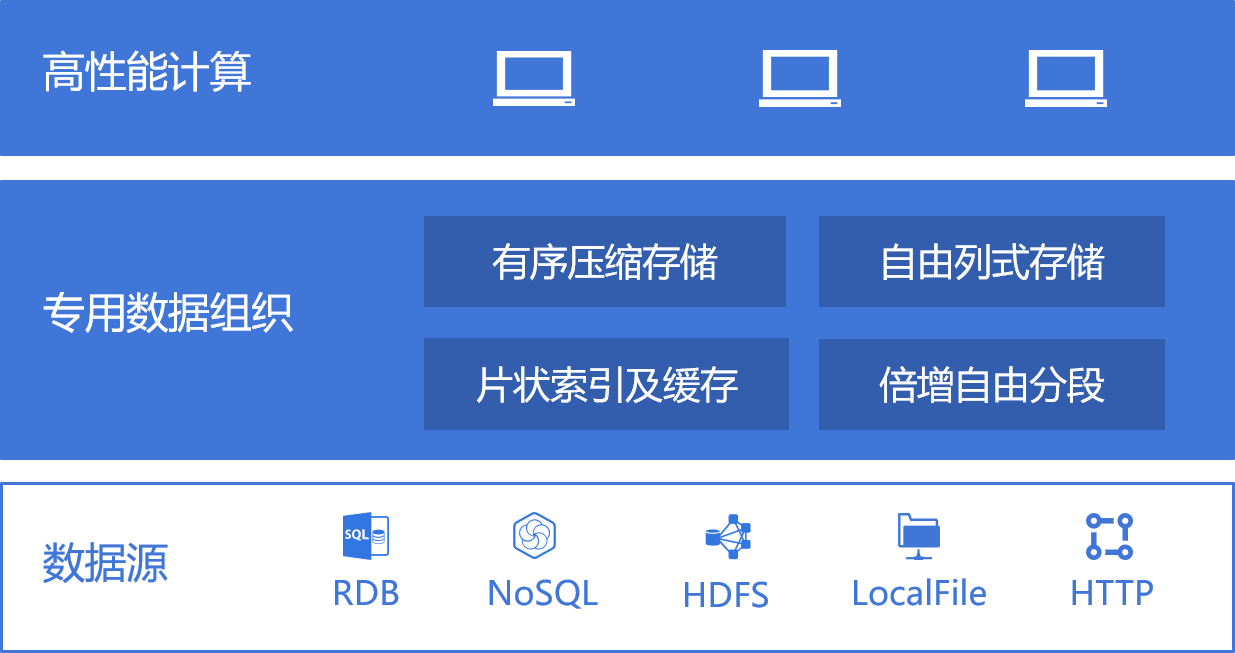集算器基于开源或数据库技术?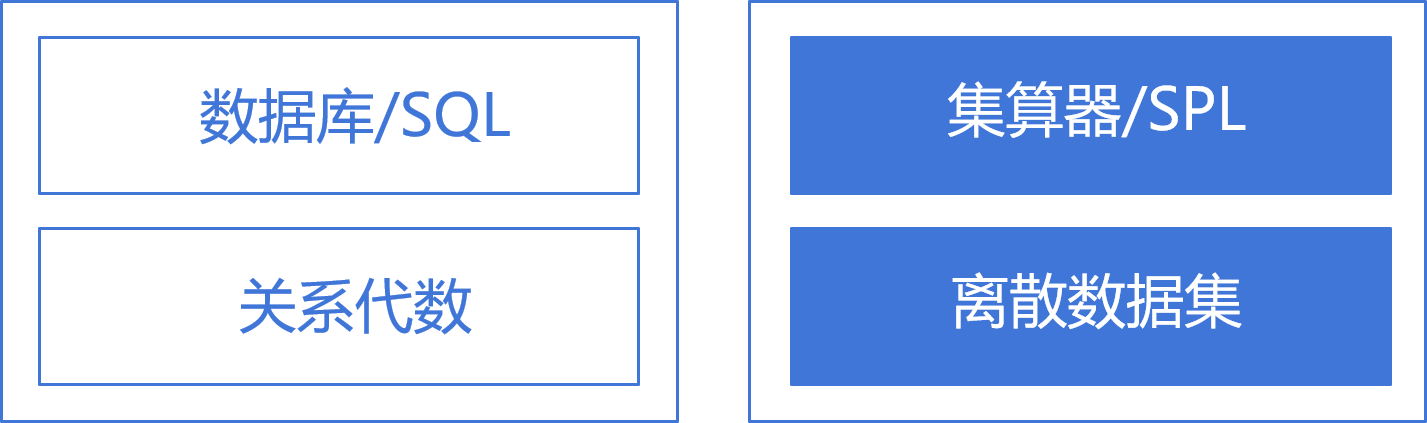集算器的学习难度如何?